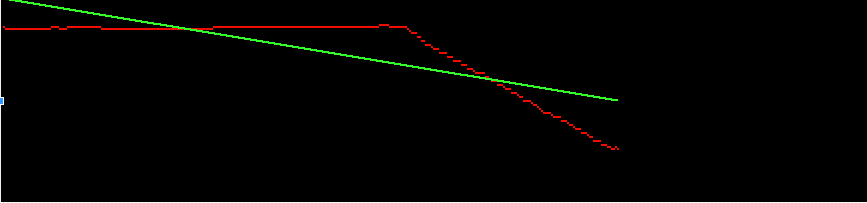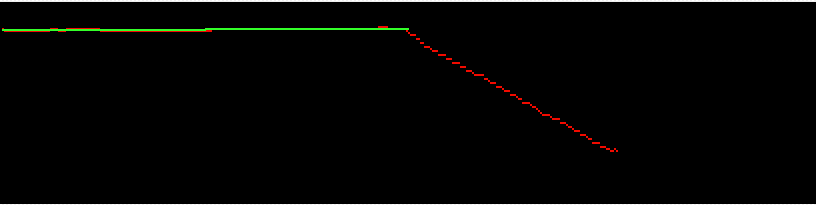## Recommended Posts

The line fitting function in NI Vision uses a robust algorithm to find a line that best fits a set of points.Although I have read the description of this VI in the NI Vision concepts manual, I am still confused about the robust algorithm it uses. So does any one know this algorithm or help to provide some references? thank you!

##### Share on other sites

The line fitting function in NI Vision uses a robust algorithm to find a line that best fits a set of points.Although I have read the description of this VI in the NI Vision concepts manual, I am still confused about the robust algorithm it uses. So does any one know this algorithm or help to provide some references? thank you!

Making Measuremnet On An Image from the Vision Concepts Manual described it in detail.

The images are broken on my browser. But it describes the NI method.

Edited by ShaunR

##### Share on other sites

Thank you, shaunR!

But I still have some confusion. For example, how does this line fitting algorithm find the initial subset of points to start, and use what method to fit a line to the subset of points. Strangely enough, if I set the iterations value to 0 or a number more than 1, this VI will return almost best fit line, but when I set this value to 1, it return a line that seems to be fitted by the standard fit method.

(the red spots represent the original edge points, and the green line is the fit line returned by this VI)Edited by jetqin

##### Share on other sites

Thank you, shaunR!

But I still have some confusion. For example, how does this line fitting algorithm find the initial subset of points to start, and use what method to fit a line to the subset of points. Strangely enough, if I set the iterations value to 0 or a number more than 1, this VI will return almost best fit line, but when I set this value to 1, it return a line that seems to be fitted by the standard fit method.

(the red spots represent the original edge points, and the green line is the fit line returned by this VI)

The main difference between the NI algorithm and most others is that it removes outliers. It calculates a "best fit" using the mean squared difference method and applies a score. If iterations is set to 0, it is finished and returns the line (as in your first image). If iterations are greater than "0" it removes an outlier and then re-calculates the MSD and re-calculates the score to see if the there is an improvement. It keeps iterating until it either reaches a certain score or until it reaches the iteration number

So in your second case, if you set the iterations to >0, the final point will be eliminated and it will decide that the first two are the "best fit". You need to have more data points to use this function effectively - the more, the better.

##### Share on other sites

The main difference between the NI algorithm and most others is that it removes outliers. It calculates a "best fit" using the mean squared difference method and applies a score. If iterations is set to 0, it is finished and returns the line (as in your first image). If iterations are greater than "0" it removes an outlier and then re-calculates the MSD and re-calculates the score to see if the there is an improvement. It keeps iterating until it either reaches a certain score or until it reaches the iteration number

So in your second case, if you set the iterations to >0, the final point will be eliminated and it will decide that the first two are the "best fit". You need to have more data points to use this function effectively - the more, the better.

Actually, the first image is the fit line returned when the iterations is 1,and the second is the results when the iterations is 0 or >1.That's why I can't understand.

jetqin

Edited by jetqin

##### Share on other sites

Hi. It looks like ShaunR is more knowledgeable in this area, so I will try to keep my foot out of my mouth. I would guess that NI would want to optimize the primitive for default use. It would seem that the default "0" automatically performs some optimizations. A non-zero value forces the primitive to actually iterate the exact number of times that you specify. When attempting to reproduce your graphs, it did not work on very low number of data points. The primitive must have some sanity checks to prevent all of the points from being removed.

##### Share on other sites

Thank you!As you said, this VI may automatically perform some optimizations when the iterations is "0".

##### Share on other sites

the following question from Jetqin still haven't been answered:

how does this line fitting algorithm find the initial subset of points to start?

below is my personal understand for the question:

Pixel Radius specifies the neighborhood pixel relationship for the initial subset of points being used.

The pixel radius defines the maximum distance allowed, in pixels, between a valid point and the estimated line. The algorithm estimates a line where at least half the points in the set are within the pixel radius. If a set of points does not have such a line, the function attempts to return the line that has the most number of valid points.

Can anybody explain the detail process of how it works?

Edited by Techie

## Join the conversation

You can post now and register later. If you have an account, sign in now to post with your account.×   Pasted as rich text.   Paste as plain text instead

Only 75 emoji are allowed.

×   Your previous content has been restored.   Clear editor

×   You cannot paste images directly. Upload or insert images from URL.

×

×